# Swiss-Knife Polynomials and Euler Numbers

Peter Luschny, May 2010

KEYWORDS: Swiss-Knife polynomials, Beta polynomials, Euler numbers, secant numbers, tangent numbers.
Concerned with sequences:  A153641, A162660, A109449, A177762, A122045, A009006, A001586, A000182, A000364, A000111.

## Notation

We will write Sn for the secant numbers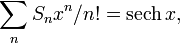$\sum_{n } S_n x^n/n! = \text{sech}\, x,$
Tn for the tangent numbers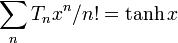$\sum_{n} T_n x^n/n! = \text{tanh}\, x$
and En for the Euler numbers$\sum_{n } E_n x^n/n! = \text{sech}\, x + \text{tanh}\, x .$
The unsigned Springer numbers are defined as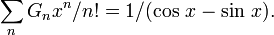$\sum_{n } G_n x^n/n! = 1/(\cos\, x - \sin\, x).$
We will make use of the convention 00 = 1.

# Swiss-Knife polynomials

## The secant Swiss-Knife polynomials

The secant Swiss-Knife polynomials are defined as$S_n(z)=\sum_{k=0}^{n}\binom{n}{k} S_k z^{n-k} .$ (I)

The first few of these polynomials are given in table 1.

 Sn(x) S0 1 S1 x S2 x2 - 1 S3 x3 - 3x S4 x4 - 6x2 + 5 S5 x5 - 10x3 + 25x S6 x6 - 15x4 + 75x2 - 61 S7 x7 - 21x5 + 175x3 - 427x

 n 0 1 2 3 4 5 6 7 Sn = Sn(0) 1 0 −1 0 5 0 −61 0 G*n = 2nSn(1/2) 1 1 −3 −11 57 361 −2763 −24611 Tn = Sn(1) 0 1 0 −2 0 16 0 −272

In table 2 we see the signed versions of the secant, the tangent and of the Springer numbers.

Shifted and scaled to (2n/n!)Sn(z/2+1/2) we get a picture of the beautiful objects of our interest (see figure 1). The polynomials have two centers´ (zero-points) at z = −1 and z = 1 which reflects the oscillating nature of the cos function.

## A recursion for the Swiss-Knife polynomials

To compute the secant Swiss-Knife polynomials Sn(z) we need to know the secant numbers Sn. If n is odd only secant numbers Sk with k < n are needed, which we assume to know by inductive hypotheses. If n is even the situation is different as then Sn <> 0. The key observation is$\text{eval}(S_n(z) - S_n, \ z=1) = -S_n(0) = - S_n \ \qquad (0 < n \ \text{even}).$

Now it is straightforward to give the recursion. In Maple parlance:

  SecPoly := proc(n,z) option remember; local k, p;
if n = 0 then 1 else p := irem(n+1,2);
z^n - p + add(if(irem(k,2) = 1, 0,
SecPoly(k,0)*binomial(n,k)*(power(z,n-k)-p)),k=2..n-1) fi end:


## The sinusoidal character of the polynomials

The scaled Swiss-Knife polynomials are defined as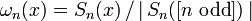$\omega_n(x) = S_n(x)\,/\,|\,S_n([n\ \text{odd}])\,|$ (II)

Plotting ωn(x) shows the sinusoidal behavior of these polynomials, which is easily overlooked in the non-scaled form. For odd index ωn(x) approximates ± sin(x π /2) and for even index ± cos(x π /2) in an interval enclosing the origin. This observation expands the observation that the Euler and Bernoulli numbers have π as a common root to an continuous scale. But much more is true: the domain of sinusoidal behavior gets larger and larger as the degree of the polynomials grows. In fact ωn(x) shows, in an asymptotic precise sense, sinusoidal behavior in the interval [-2n/πe, 2n/πe].

From these observations follows the regular behavior of the real roots of the Swiss-Knife polynomials. For example the roots of ωn(x) are close to the integer lattices: {±0, ±2, ±4, ...} if n is odd and {±1, ±3, ±5, ...} if n is even.

## The tangent Swiss-Knife polynomials

The tangent Swiss-Knife polynomials are defined as$T_n(z) = \sum_{k=0}^{n-1} \binom{n}{k} T_{n-k} z^{k} .$ (III)

The first few of these polynomials are given in table 3.

 Tn(x) T0 0 T1 1 T2 2x T3 3x2 - 2 T4 4x3 - 8x T5 5x4 - 20x2 + 16 T6 6x5 - 40x3 + 96x T7 7x6 - 70x4 + 336x2 - 272

 n 0 1 2 3 4 5 6 7 Tn = Tn(0) 0 1 0 −2 0 16 0 −272 G*n = (−1)n+2nTn(-1/2) 1 1 −3 −11 57 361 −2763 −24611 Sn = 1 − Tn(1) 1 0 −1 0 5 0 −61 0

In table 4 we see the signed versions of the secant, the tangent and of the Springer numbers.

Scaled to (2n/ n!)Tn(z) we get a nice plot of these polynomials (see figure 2). The polynomials have one center´ at z = 0 which reflects the behavior of tanh in the neighborhood of 0.

## Putting things together

Let us defined the sum of the S and T Swiss-Knife polynomials, weighted by p and q, which we assume to be complex values defined in a superset of the interval (-1, 1).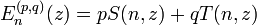$E_n^{(p,q)}(z) = pS(n,z) + qT(n,z)$ (IV)
 En(p,q) (z) E0 p E1 pz + q E2 pz2+ 2zq − p E3 pz3 + 3z2q - 3pz - 2q E4 pz4 + 4z3q - 6pz2 - 8zq + 5p E5 pz5 + 5z4q - 10pz3 - 20z2q + 25pz + 16q E6 pz6 + 6z5q - 15pz4 - 40z3q + 75pz2 + 96zq - 61p

We call these polynomials the pq-Swiss-Knife polynomials. The special case En(z) = En(1,1)(z) could be called Euler polynomials´ if this name would be at our disposal.

By comparison, the conventional Euler polynomials are, despite their name, not very nice polynomials -- they have rational coefficients whereas the Sn(z), Tn(z) and our En(z) polynomials have integer coefficients and can be added much easier to the OEIS. However, our polynomials share many of the features of the Euler polynomials. So if you use the traditional Euler polynomials consider also the Swiss-Knife polynomials. They come for a better price and might also do the job. We give an example in the next section.

The special choice of p and q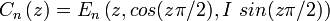$C_{n} \left(z\right) = E_{n} \left(z, cos(z \pi /2), I \ sin(z \pi /2) \right)$

leads to the complex Swiss-Knife polynomials.

## Sum of alternating powers

The Swiss-Knife polynomials provide a general formula for alternating sums of powers similar to the formula which are provided by the Bernoulli polynomials for non-alternating sums of powers.

• Alternating sum of powers of odd integers (n ≥ 0)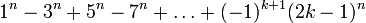$1^n-3^n+5^n-7^n+\ldots+(-1)^{k+1}(2k-1)^n$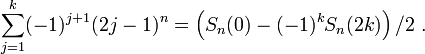$\sum_{j=1}^{k} (-1)^{j+1}(2j-1)^n =\left(S_n(0) - (-1)^k S_n(2k) \right) /2 \ .$

• Alternating sum of powers of even integers (n ≥ 1)$2^n-4^n+6^n-8^n+\ldots+(-1)^{k+1}(2k)^n$$\sum_{j=1}^{k} (-1)^{j+1}(2j)^n = \left(S_n(1)-(-1)^k S_n(2k+1) \right) /2 \ .$

• One formula for both cases: ε in {0, 1}$\sum_{j=1}^{k} (-1)^{j+1}(2j-1+\epsilon)^n = \left(S_n(\epsilon)- (-1)^k S_n(2k+\epsilon) \right) /2 \ .$

• In particular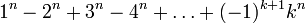$1^n-2^n+3^n-4^n+\ldots+(-1)^{k+1}k^n$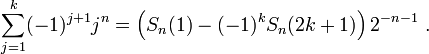$\sum_{j=1}^{k} (-1)^{j+1} j^n = \left(S_n(1)- (-1)^k S_n(2k+1) \right) 2^{-n-1} \ .$

Note that all this can be done with integer arithmetic only. Even an evaluation with Euler polynomials has to use rational arithmetic because the coefficients of the Euler polynomials are rational numbers -- in contrast to the coefficients of the Swiss-Knife polynomials which are integers. Sequences covered by this formula include

A001057, A062393, A062392, A011934, A144129, A077221, A137501, A046092.

## Short guide to the relevant sequences at OEIS

The secant, tangent and Euler numbers are core sequences of OEIS, can be easily found and have a rich corpus of comments. Therefore I will restrict myself here to the afore-mentioned polynomials.

The secant Swiss-Knife polynomials Sn(x) are in A153641. They are denoted there Wn(x) and introduced via the double-sum transformation applied to the Kwang-Wu Chen sequence. Peter Bala gives the representation Sn(x) = -2/(n+1)B(X;n+1,x), thereby associating them with certain generalized Bernoulli polynomials.

The tangent Swiss-Knife polynomials Tn(x) are in A162660. They are denoted there Vn(x), called complementary Swiss-Knife polynomials and introduced via a double-sum transformation which will be discussed below. Also a formula is given expressing the polynomials as a binomials sum with secant numbers. Comparing this formula with our definition here gives the identity$\sum_{k=0}^{n-1}\binom{n}{k} T_{n-k} z^{k} = -z^n + \sum_{k=0}^{n} \binom{n}{k} S_{k} (z+1)^{n-k}$

The En(1,1)(z) polynomials are in A109449, albeit with unsigned coefficients and in ascending order of powers. Again both a representation in terms of Euler polynomials as well in terms of the afore-mentioned transformation is given.

The definitions used here are streamlined compared to those on OEIS. Moreover the computational relations used here give a more intuitive and compact framework, building on a single recursion, described in the  appendix.

## A common representation

We now give a representation of the secant, tangent and Euler numbers based on a general sequence-to-sequence transformation.

Given a sequence F the transform of F is defined as Γ(F)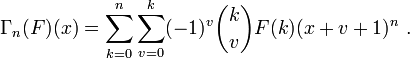$\Gamma_n(F)(x) = \sum_{k=0}^{n}\sum_{v=0}^{k}(-1)^{v} \binom{k}{v}F(k)(x+v+1)^n \ .$ (V)

•  Secant polynomials Sn(x) = Γn(σ)(x)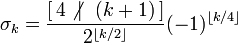$\sigma_k = \frac{[\,4\not{ \mid} \ \ (k+1)\,]}{2^{\lfloor k / 2 \rfloor}} {(-1)^{\lfloor k/4 \rfloor }}$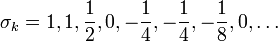$\sigma_k = 1,1,\frac{1}{2},0,-\frac{1}{4},-\frac{1}{4},-\frac{1}{8},0,\ldots$

•  Tangent polynomials Tn(x) = Γn(τ)(x), τ0 = 0 and for k > 0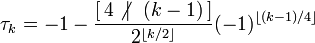$\tau_k = -1 -\frac{[\,4\not{ \mid} \ \ (k-1)\,]}{2^{\lfloor k / 2 \rfloor}} (-1)^{\lfloor (k-1)/4 \rfloor }$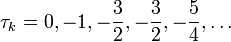$\tau_k= 0,-1,-\frac{3}{2},-\frac{3}{2},-\frac{5}{4},\ldots$

•  E polynomials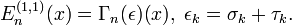$E^{(1,1)}_n(x) = \Gamma_n(\epsilon)(x), \ \epsilon_k= \sigma_k + \tau_k.$

## Summary

The Swiss-Knife polynomials are closely related to the family of Euler-Bernoulli polynomials and numbers. They come in three flavors. The secant, the tangent and the E Swiss-Knife polynomials are defined via the exponential generating functions

   s(x,t) = exp{xt} sech(t);
t(x,t) = exp{xt} tanh(t);
e(x,t) = exp{xt}(sech(t) + tanh(t)).


The coefficients of the polynomials are integers, in contrast to the coefficients of the Euler and Bernoulli polynomials, which are rational numbers (see HMF, page 809). Thus the Swiss-Knife polynomials can be computed more easily and applied to special number theory.

The Euler, Bernoulli, Genocchi, Euler zeta, tangent as well as the up-down numbers and the Springer numbers are either values or scaled values of these polynomials. The Swiss-Knife polynomials are also connected with the Riemann and Hurwitz zeta function. They display a strong and beautiful sinusoidal behavior if properly scaled which has its basis in the Fourier analysis of the generalized zeta function.

The first time I considered the Swiss-Knife polynomials was on March 2008. I was surprised that I could not find a discussion of these polynomials in the literature. Therefore I added a page describing the polynomials to the English Wikipedia. This page was deleted on (2008-12-27) from Wikipedia on the ground that the Wikipedia editors "strongly suggest that the subject of the article is original research". (Original research is not allowed to be published on Wikipedia.) If you know an earlier mention in the literature please let me know.

# Beta polynomials

## Beta polynomials and Euler numbers

Let us define the Dirichlet β function as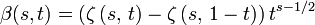$\beta(s,t)=\left(\zeta \left(s,\,t\right)-\zeta \left(s,\,1-t\right)\right)t^{s-1/2}$

Note that our definition is slightly different from the standard definition. We will also use the notation β(s) = β(s, 1/4).

   beta := s -> 2*4^(-s)*(Zeta(0,s,1/4)-Zeta(0,s,3/4));


The β polynomials are defined as β0(z) = 1 and for n > 0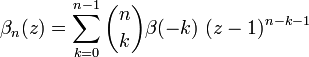$\beta_n(z) = \sum_{k=0}^{n-1}\binom{n}{k}\beta(-k)\ (z-1)^{n-k-1}$ (VI)

In my last blog (Zeta Polynomials and Harmonic Numbers, April 2010) I used a similar definition with β(-k) replaced by ζ(-k) to introduce the ζ-polynomials which led to a representation of the Bernoulli numbers.

A recursion for the β-polynomials is β0(z) = 1 and for n > 0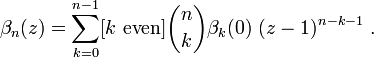$\beta_n(z) = \sum_{k=0}^{n-1}[ k \ \text{even} ] \binom{n}{k}\beta_k(0)\ (z-1)^{n-k-1} \ .$ (VII)

The first few β-polynomials are given in table 6.

 β0(z) 1 β1(z) 1 β2(z) z − 1 β3(z) z2 − 2z − 2 β4(z) z3 − 3z2 − 3z + 5 β5(z) z4 − 4z3 − 4z2 + 16z + 16 β6(z) z5 − 5z4 − 5z3 + 35z2 + 35z − 61

Some values of the β polynomials can be found in table 7.

 n 0 1 2 3 4 5 6 7 8 9 10 z = 0 1 1 −1 −2 5 16 −61 −272 1385 7936 −50521 z = 1 1 1 0 −1 0 5 0 −61 0 1385 0

We see the signed Euler numbers En =  βn(0). The unsigned Euler  numbers count the alternating permutations of {1, 2, 3, ..., n}.

Note that this terminology is in accordance with R. P. Stanley's recent "A Survey of Alternating Permutations" (preliminary version of 21 September 2009), who gives the definition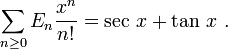$\sum_{n \ge 0} E_n \frac{x^n}{n!} = \sec \, x + \tan \, x \ .$

And, believe it or not, even Don Knuth changed his Euler numbers in Concrete Mathematics: "I'm changing the notation for Euler numbers to agree with papers in combinatorics ...". (See the Errata page of CM.)

Now, if we take the transition from the secant numbers to the full-fledged Euler numbers (secant plus tangent) as suggested by Richard Stanley's definition of the Euler numbers seriously, is it then not natural to consider also 'new' Euler polynomials which reflect this change?

What polynomials would be more appropriate for that? The β polynomials or the E(1,1)(n, z) Swiss-Knife polynomials? What is the relation between the β polynomials and the Swiss-Knife polynomials anyway? We will come back to this question after an important message by our sponsors Euler and Catalan.

## Some zeta and beta values

Consider the following function$EC(n,z) = \beta_n(z)\frac{(\pi/2)^{n+1}}{\Gamma(n+1)}t(n) .$ (VIII)

Here t(x) is defined as t(x) = cos(πx/2)/2 + sin(πx/2)/(2 − 2−x)

For z = 0 we get the famous Euler-Catalan formulas. Once upon a time these formulas and the proof that their values are rational multiples of some power of π belonged to the 1 million dollar problems in mathematics.

Maple computes EC(n, 0) for n = 0 .. 7 as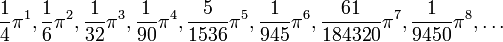$\frac{1}{4}\pi^1, \frac{1}{6}\pi^2, \frac{1}{32}\pi^3, \frac{1}{90}\pi^4, \frac{5}{1536}\pi^5, \frac{1}{945}\pi^6, \frac{61}{184320} \pi^7, \frac{1}{9450}\pi^8, \ldots$

By the standard definitions of the ζ and β functions$\zeta(s) = \sum_{n=0}^\infty \frac{1} {(n+1)^s} \ , \ \beta(s) = \sum_{n=0}^\infty \frac{(-1)^n} {(2n+1)^s}$

we see that this is the sequence$\beta(1),\ \zeta(2),\ \beta(3),\ \zeta(4),\ \beta(5),\ \zeta(6),\ \beta(7),\ \zeta(8), \ \ldots$

Our approach combines these important special cases of the ζ and β function in a uniform way by only referring to the beta polynomials.

## Beta polynomials and Swiss-Knife polynomials

I am quite fond of the β polynomials. However, I think the β polynomials are just the Swiss-Knife polynomials in disguise. Given the secant Swiss-Knife polynomials this is how we can arrive at the β polynomials by a  formal procedure:

Step 1: Lower the degree of the monomials by 1.
Step 2: Set constant / x to 0.
Step 3: Substitute x by z − 1.

For example S_6(x) = x^6-15x^4+75x^2-61.

After step 1: x^5-15x^3+75x-61/x.
After step 2: x^5-15x^3+75x.
After step 3: (z-1)^5-15(z-1)^3+75(z-1).

Expanding gives z^5-5z^4-5z^3+35z^2+35z-61, which is β_6(z).


Funny how the deleted -61 reappeared! Euler numbers just pop up everywhere.

# Appendix

## A Maple session

# This worksheet gives a recursion
# for the pq-Swiss-Knife polynomials

pqSwissKnifePoly := proc(n,z,p,q)
local P,Q,SECH,TANH,power,eps,BetaPoly,SecPoly,TanPoly;

# teach Maple 0^0 = 1
power := proc(u,v) if(u=0 and v=0,1,u^v) end:

BetaPoly := proc(n, z) option remember; local k;
if n = 0 then 1 else add(if(k mod 2 = 1, 0,
binomial(n,k)*BetaPoly(k,0)*power(z-1,n-k-1)),
k = 0..n-1) fi end:

SECH := n -> if(n mod 2=1,0,BetaPoly(n,0)):
TANH := n -> if(n mod 2=0,0,BetaPoly(n,(n+1) mod 2)):

SecPoly := proc(n,z) local k;

TanPoly := proc(n,z) local k;

P := if(p = 0, 0, SecPoly(n,z));
Q := if(q = 0, 0, TanPoly(n,z));
p*P + q*Q end:

# -----------------------------------------------------------

showPoly := proc(z,p,q) local i;
seq(print(sort(expand(pqSwissKnifePoly(i,z,p,q)))),i=0..7)end;

showPoly(z,p,q); # pqSwissKnife polynomials
showPoly(z,1,0); # SecSwissKnife polynomials
showPoly(z,0,1); # TanSwissKnife polynomials
showPoly(z,1,1); # EulerSwissKnife polynomials
showPoly(z,cos(z*Pi/2),I*sin(z*Pi/2)); # complex SwissKnife

seq(pqSwissKnifePoly(i,0,1,0),i=0..12); # Secant numbers
seq(pqSwissKnifePoly(i,0,0,1),i=0..12); # Tangent numbers
seq(pqSwissKnifePoly(i,0,1,1),i=0..12); # Euler numbers

# Springer numbers (signed)
seq(  2^i*pqSwissKnifePoly(i,1/2,1,0),i=0..10);
seq(1-2^i*pqSwissKnifePoly(i,1/2,0,1),i=0..10);

# Generalized Euler numbers (signed)
seq(1-2^i*pqSwissKnifePoly(i, 1/2,1,1),i=0..10);
seq(1-2^i*pqSwissKnifePoly(i,-1/2,1,1),i=0..10);

Note the difference between Springer numbers (generalized
secant/tangent numbers) and generalized Euler numbers!
This gets often confused.

EulerCatalan := proc(n,z) local t;
t := x -> cos(Pi*x/2)/2 + sin(Pi*x/2)/(2-2^(-x));
pqSwissKnifePoly(n,z,1,0)*t(n)*(Pi/2)^(n+1)/GAMMA(n+1) end:

seq(EulerCatalan(i, i mod 2), i = 0..7);


The following little routine computes five famous sequences of special numbers in mathematics (Euler, Tangent, Springer, Genocchi, Bernoulli) in a few lines, using only the power function and the binomial coefficients. It is based on an evaluation of a representation of the secant, tangent and E polynomials which we have discussed above.

SwissKnifeDecompositions := proc(type, n) local W,w,x,y;

W := proc(n,k) local v, pow;
pow := (a,b) -> if a = 0 and b = 0 then 1 else a^b fi;
if irem(k+1,4) = 0 then 0 else (-1)^iquo(k+1,4)*2^(-iquo(k,2))
fi end;

w := proc(n) local k; print(seq(W(n,k),k=0..n));

if type = "eul" then x:=0;   y:=1;   w(n)
elif type = "tan" then x:=1;   y:=1;   w(n)
elif type = "spr" then x:=1/2; y:=2^n; w(n)
elif type = "gen" then x:=-1;  y:=n+1; w(n)/2^n
elif type = "ber" then x:=1;   y:=n+1; w(n)/(4^(n+1)-2^(n+1))
fi end:

# Let us check the function:
types := ["eul", "tan", "spr", "gen", "ber"]:
for t in types do seq(SwissKnifeDecompositions(t,i),i=0..8) od;
`

## New sequences for OEIS

Surprisingly the (coefficients of the) β polynomials are not yet in OEIS. Therefore I submitted these numbers to the OEIS A177762.